Existence of Z-Transform

# Existence of Z-Transform - Notes | Study Digital Signal Processing - Electrical Engineering (EE)

 1 Crore+ students have signed up on EduRev. Have you?

A system, which has system function, can only be stable if all the poles lie inside the unit circle. First, we check whether the system is causal or not. If the system is Causal, then we go for its BIBO stability determination; where BIBO stability refers to the bounded input for bounded output condition.

This can be written as;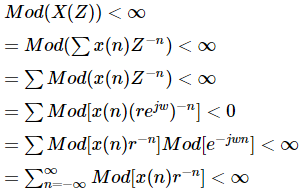The above equation shows the condition for existence of Z-transform.

However, the condition for existence of DTFT signal is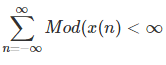Example 1

Let us try to find out the Z-transform of the signal, which is given as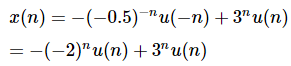Solution − Here, for −(−2)nu(n) the ROC is Left sided and Z<2

For 3nu(n) ROC is right sided and Z>3

Hence, here Z-transform of the signal will not exist because there is no common region.

Example 2

Let us try to find out the Z-transform of the signal given by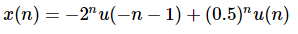Solution − Here, for −2nu(−n−1) ROC of the signal is Left sided and Z<2

For signal (0.5)nu(n)ROC is right sided and Z>0.5

So, the common ROC being formed as 0.5<Z<2

Therefore, Z-transform can be written as;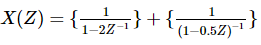Example 3

Let us try to find out the Z-transform of the signal, which is given as x(n) = 2r(n)

Solution − r(n) is the ramp signal. So the signal can be written as;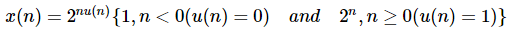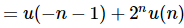Here, for the signal u(−n−1) and ROC Z<1 and for 2nu(n) with ROC is Z>2.

So, Z-transformation of the signal will not exist.

Z -Transform for Causal System

Causal system can be defined as h(n) = 0,n < 0. For causal system, ROC will be outside the circle in Z-plane.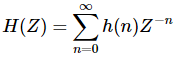Expanding the above equation,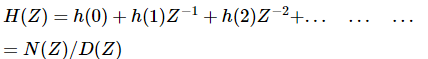For causal systems, expansion of Transfer Function does not include positive powers of Z. For causal system, order of numerator cannot exceed order of denominator. This can be written as-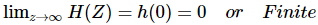For stability of causal system, poles of Transfer function should be inside the unit circle in Z-plane.

Z-transform for Anti-causal System

Anti-causal system can be defined as h(n) = 0,n ≥ 0. For Anti causal system, poles of transfer function should lie outside unit circle in Z-plane. For anti-causal system, ROC will be inside the circle in Z-plane.

The document Existence of Z-Transform - Notes | Study Digital Signal Processing - Electrical Engineering (EE) is a part of the Electrical Engineering (EE) Course Digital Signal Processing.
All you need of Electrical Engineering (EE) at this link: Electrical Engineering (EE)

## Digital Signal Processing

3 videos|50 docs|54 tests
 Use Code STAYHOME200 and get INR 200 additional OFF

## Digital Signal Processing

3 videos|50 docs|54 tests

Track your progress, build streaks, highlight & save important lessons and more!

,

,

,

,

,

,

,

,

,

,

,

,

,

,

,

,

,

,

,

,

,

;